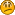Hello Guest

• 6 Replies
• 12842 Views####Daslee

•• 126« on: July 01, 2012, 12:12:46 »
Hello. Im creating little physics for 2d games, now im testing it in simple 2d double buffered window. I have gravity for quads, and I created mass variable, but I just do not know where to use mass. Here is my code increasing y velocity with gravity:
Code: [Select]
`if(wQuad.falling || wQuad.jumping){ wQuad.yVel += GRAVITY * TIME_STEP;}`
But I do not know formula of gravity with mass, does it must be multiplied with gravity, or time_step or maybe it must be added to time_step or gravity. I do not know.EDIT: Fixed, mass must be multiplied with gravity. Source: http://hyperphysics.phy-astr.gsu.edu/hbase/mass.html (m * g)
« Last Edit: July 02, 2012, 08:55:35 by Daslee »####CodeBunny

•• 562##### Re: Adding mass to gravity
« Reply #1 on: July 01, 2012, 13:19:43 »
Ummm... Mass should not be involved in most gravity equations. Rather, it should, but in an earthlike situation it has an absolutely negligible effect, and all objects get accelerated by the gravitational constant.

Gravity is two objects with mass pulling on each other. In everyday life, we only notice the earth pulling on individual objects. That's because of this equation:

(force applied by gravity) = (mass of object 1) * (mass of object 2) * (coefficient of gravity) / (distance between objects squared)

Since acceleration applied to an object is (force applied) / (mass of object), gravitic acceleration (what we are interested in), is simply:

(acceleration from gravity) = (mass of other object) * (coefficient of gravity) / (distance between objects squared)

In planetary situations, we simplify the scenario by having a steady rate of gravitic acceleration. On earth, that's 9.84 m/s.

So, in a game, just accelerate your objects by a steady rate. Leave mass out of it.

In summary: leave mass out of your gravity accelerations; it's useful for momentum and force equations instead.####Daslee

•• 126##### Re: Adding mass to gravity
« Reply #2 on: July 01, 2012, 13:35:39 »
I can't understand, I readed your post 2 times, but I still do not know how to do that in game coding. :/####CodeBunny

•• 562##### Re: Adding mass to gravity
« Reply #3 on: July 01, 2012, 13:50:53 »
Object's velocity = [vX, vY]
Gravity is pulling the object in the -Y direction.

For every update:

Object's Velocity.vY -= (gravity * (amount of seconds since last update))####Daslee

•• 126##### Re: Adding mass to gravity
« Reply #4 on: July 01, 2012, 15:16:26 »
Quote
amount of seconds since last update
How I can get that? Thread.sleep miliseconds?####CodeBunny

•• 562##### Re: Adding mass to gravity
« Reply #5 on: July 02, 2012, 00:27:45 »
Uhhh... you should have a central game loop for updating/rendering, and you should be detecting that there. Plus, it should NOT be based on Thread.sleep (it's horribly inaccurate sometimes), and instead be based on LWJGL's Display.sync().####Daslee

•• 126##### Re: Adding mass to gravity
« Reply #6 on: July 02, 2012, 08:58:02 »
I see it's very difficult to do it. So I better lock this thread, maybe someone need that formulas which you posted, so better do not delete.